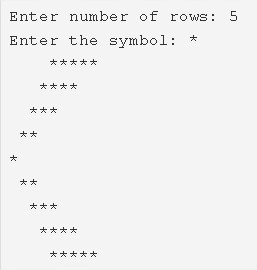# Left Arrow Star Pattern Program in C | C Programs

C program to print the left arrow star pattern – In this article, we will summarise in on the different methods to print a left arrow star pattern in C programming.

Suitable examples and sample programs have also been added so that you can understand the whole thing very clearly. The compiler has also been added with which you can execute it yourself.

The ways used in this piece are as follows:

• Using For Loop
• Using While Loop
• Using Do-While Loop

As the name of the heading suggests, you need to print a left arrow star pattern.As it is implied by the photo, firstly, you need to enter the number of rows. Then, the arrow will be printed accordingly.

Thus, the various ways to do so are as follows:

## Using For Loop

1. Read the entered rows number, entered symbol and store the number into the variable n, symbol into the variable ch.

2) The 1st for loop iterates through rows with the structure for(i=1,i<=n;i++).

a) The 2nd for loop iterates through columns with the structure for(j=1;j<=n-i;j++).

This loop prints space.

b) The 3rd for loop iterates through columns with the structure for(j=i;j<=n;j++).

This loop prints symbol

After above steps, the upper part of the left arrow star pattern will be printed.

c) Cursor comes to next line for each iteration of i.

3) The 4th for loop with the structure for(i=1;i<n;i++) iterates through rows.

a) The 5th for loop with the structure for(j=0;j<i;j++) iterates through columns.

This loop prints space.

b) The 6th for loop iterates through columns with the structure for(j=0;j<=i;j++).

This loop prints symbol.

c) cursor comes to next line for each iteration of i.

After the above steps, the lower part of the left arrow star pattern will be printed.

Output:

### Using While Loop

1. The 1st while loop with the structure i<=n iterates through rows until the condition becomes false.

a) j=1, The 2nd while loop iterates through columns until the condition j<=n-i becomes false.

This loop Prints space.j value increased by 1.

b) j=i, The 3rd while loop iterates through columns until the condition j<=n fails.

This loop prints the symbol which is entered by the user.j value increased by 1.

c) Cursor comes to next line.i value increased by 1.

After the above steps, we will get half part of the left arrow star pattern.

2) i=1, The 4th while loop iterates through rows until the condition i<n becomes false.

a) j=0, The 5th while loop iterates through columns until the condition j<i fails.

This loop prints space.j value increased by 1.

b) j=0, The 6th while loop iterates through columns until the condition j<=i fails.

This loop prints symbol.j value increased by 1.

c) Cursor comes to next line.i value increased by 1.

From the above steps, we will get next half part of the left arrow star pattern.

Output:

#### Using Do-while Loop

1. i=1, The 1st do-while loop iterates through rows.

a) j=1, The 2nd do-while loop iterates through columns.

This loop prints space.j value increased by 1.

Checks the condition j<=n-i.If the condition is true then loop iterates again.Repeats until the condition becomes false.

b) j=i, The 3rd do-while loop iterates through columns.

Prints symbol.j value increased by 1.

Checks the condition j<=n.If the condition is true then loop iterates again.Repeats until the condition becomes false.

c) Cursor comes to next line.i value increased by 1.Checks the condition i<=n. If the condition is true then loop iterates again.Repeats until the condition becomes false.

By the above steps, we will get the half part of the left arrow star pattern.

2) i=1, The 4th do-while loop iterates through rows.

a) j=0, the 5th do-while loop iterates through columns.

Prints space.j value increased by 1.

Checks the condition j<i.If the condition is true then loop iterates again.Repeats until the condition becomes false.

b) j=0, the 6th do-while loop iterates through columns.

Prints symbol.j value increased by 1.

Checks the condition j<=i.If the condition is true loop iterates again. Repeats until the condition becomes false.

c) Cursor comes to next line.i value increased by 1.Checks the condition i<n. If the condition is true then loop iterates again.Repeats until the condition becomes false.

From the above steps, we will get remaining half part of the left arrow star pattern.

Output:
x

## C Program To Print Number Of Days In A Month | Java Tutoring

C program to input the month number and print the number of days in that ...